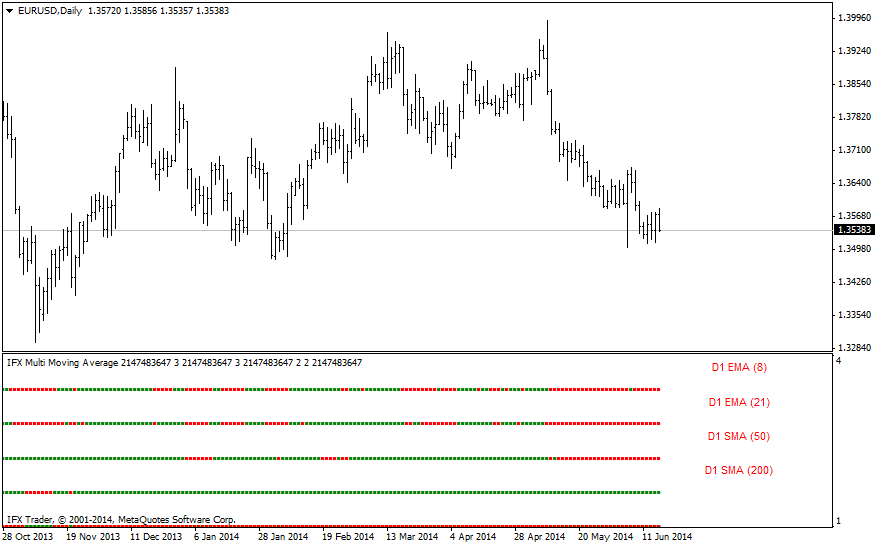#### 公式

SMA = Sum(Price)/n, where

Price — price at the moment from 1 to n;

n — moving average period.

#### 交易应用MA1 = 13

MA2 = 34

MA3 = 55

MA4 = 89